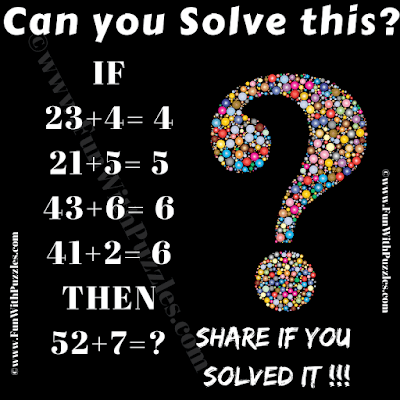This is a tough logical reasoning puzzle question for genius brains. In this puzzle question, you will see some logical number equations. Your challenge in this logical reasoning puzzle question is to crack the logic in this puzzle and then find the value of the missing number. Let's see if you solve this genius maths logic puzzle?Can you find the value of the missing number?
The answer to this "Only for Genius Logical Reasoning Puzzle Question", can be viewed by clicking on the answer button.

syedshan said...

According to this logic, the first equation should be equal to -4 not 4.

Rajesh Kumar said...

@ Syedshan
Yes, you are right. I have corrected the answer logic for this puzzle.

Damodar Adhikari , The BETA Park Thimphu, Bhutan 🇧🇹 said...

D= abc/ab also is a possible logic ,

With this we get 52+7 as 7

Vinny O. said...

The answer I got is 21. By taking the absolute value of difference between the first two two numbers in each equation
and then multiplying that by the third number you get your product.
Ex. |2-3|4=4
|2-1|5=5
|4-3|6=6
|4-1|2=6
So
|5-3|7=21